# Floating Point Arithmetic

Floating point (FP) representations of decimal numbers are essential to scientific computation using scientific notation. The standard for floating point representation is the IEEE 754 Standard. In a computer, there is a tradeoff between range and precision – given a fixed number of binary digits (bits), precision can vary inversely with range. In this section, we overview decimal to FP conversion, MIPS FP instructions, and how registers are used for FP computations.

We have seen that an n-bit register can represent unsigned integers in the range 0 to 2n-1, as well as signed integers in the range -2n-1 to -2n-1-1. However, there are very large numbers (e.g., 3.15576 · 1023), very small numbers (e.g., 10-25), rational numbers with repeated digits (e.g., 2/3 = 0.666666…), irrationals such as 21/2, and transcendental numbers such as e = 2.718…, all of which need to be represented in computers for scientific computation to be supported.

We call the manipulation of these types of numbers floating point arithmetic because the decimal point is not fixed (as for integers). In C, such variables are declared as the `float` datatype.

#### 3.4.1. Scientific Notation and FP Representation

Scientific notation has the following configuration:and can be in normalized form (mantissa has exactly one digit to the left of the decimal point, e.g., 2.3425 · 10-19) or non-normalized form. Binary scientiic notation has the folowing configuration, which corresponds to the decimal forms: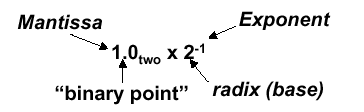Assume that we have the following normal format for scientific notation in Boolean numbers:

+1.xxxxxxx2 · wyyyyy2 ,

where “xxxxxxx” denotes the significand and “yyyyy” denotes the exponent and we assume that the number has sign S. This implies the following 32-bit representation for FP numbers: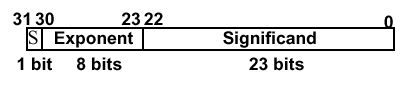which can represent decimal numbers ranging from -2.0 · 10-38 to 2.0 · 1038.

#### 3.4.2 Overflow and Underflow

In FP, overflow and underflow are slightly different than in integer numbers. FP overflow (underflow) refers to the positive (negative) exponent being too large for the number of bits alloted to it. This problem can be somewhat ameliorated by the use of double precision, whose format is shown as follows: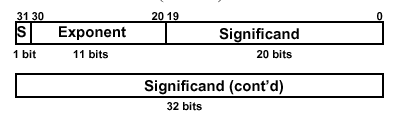Here, two 32-bit words are combined to support an 11-bit signed exponent and a 52-bit significand. This representation is declared in C using the `double` datatype, and can support numbers with exponents ranging from -30810 to 30810. The primary advantage is greater precision in the mantissa.

The following chart illustrates specific types of overflow and underflow encountered in standard FP representation: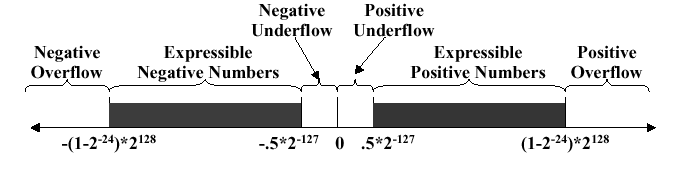### 3.5. Floating Point in MIPS

The MIPS FP architecture uses separate floating point insturctions for IEEE 754 single and double precision. Single precision uses `add.s`, `sub.s`, `mul.s`, and `div.s`, whereas double precision instructions are `add.d`, `sub.d`, `mul.d`, and `div.d`. These instructions are much more complicated than their integer counterparts. Problems with implementing FP arithmetic include inefficiencies in having different instructions that take significantly different times to execute (e.g., division versus addition). Also, FP operations require much more hardware than integer operations.

Thus, in the spirit of RISC design philosophy, we note that (a) a particular datum is not likely to change its datatype within a program, and (b) some types of programs do not require FP computation. Thus, in 1990, the MIPS designers decided to separate the FP computations from the remainder of the ALU operations, and use a separate chip for FP (called the coprocessor). A MIPS coprocessor contains 32 32-bit registers designated as `\$f0`, `\$f1`, …, etc. Most of these registers are specified in the `.s` and `.d` instructions. Double precision operands are stored in register pairs (e.g., `\$f0,\$f1` up to `\$f30,\$f31`).

The CPU thus handles all the regular computation, while the coprocessor handles the floating point operations. Special instructions are required to move data between the coprocessor(s) and CPU (e.g., `mfc0`, `mtc0`, `mfc0`, `mtc0`, etc.), where cn refers to coprocessor #n. Similarly, special I/O operations are required to load and store data between the coprocessor and memory (e.g., `lwc0`,`swc0`, `lwc1`, `swc1`, etc.)

FP coprocessors require very complex hardware, as shown in Figure 3.23, which portrays only the hardware required for addition.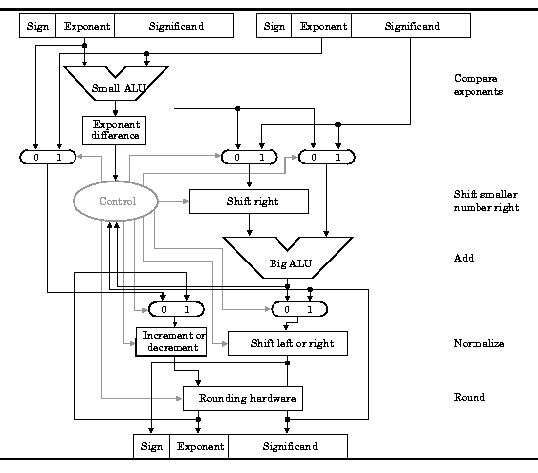Here, we assume that there is a coprocessor c1 connected to the CPU. The values 5.0 and 9.0 are respectively loaded into registers `\$f16` and `\$f18` using the `lwc1` instruction with the global pointer as base address and the variables `const5` and `const9` as offsets. The single precision division operation puts the quotient of 5.0/9.0 into `\$f16`, and the remainder of the computation is straightforward. As in all MIPS procedure calls, the `jr` instruction returns control to the address stored in the `\$ra` register.